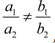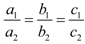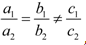Visitors Online: 77 | Thursday 14th November 2019

CBSE Subject Notes

CBSE Guess > eBooks > Class IX > Maths > Linear Equations in Two Variables By Chitra Studies

Maths (Linear Equations)

Introduction to Linear Equations in Two Variables

Linear equation in two variable: An equation in the form ax + by + c = 0, where a, b and c are real numbers, and a and b are not both zero (a2 + b2 ≠ 0), is called a linear equation in two variables x and y.

Solution of a linear equation in two variables: Every solution of the equation is a point on the line representing it.
Each solution (x, y) of a linear equation in two variables, ax + by + c = 0, corresponds to a point on the line representing the equation, and vice versa.

General form of pair of linear equations in two variables:
The general form for a pair of linear equations in two variables x and y is
a1x + b1y + c1 = 0 and
a2x + b2y + c2 = 0,
Where a1, b1, c1, a2, b2, c2 are all real numbers and a12 + b12 ≠ 0, a22 + b22 ≠ 0.

Geometrical representation of pair of linear equations in two variables
The geometrical representation of a linear equation in two variables is a straight line.

Pair of linear equations in two variables:
If we have two linear equations in two variables in a plane, and we draw lines representing the equations, then:

 Condition Result Lines intersecting at a single point => The pair of equations has a unique solution. The pair of linear equations is consistent Lines parallel to each other => No solutions. The pair of linear equations is inconsistent. Coincident lines => Infinite number of solutions. The pair of linear equations is consistent and dependent.

Algebraic interpretation of pair of linear equations in two variables
The pair of linear equations represented by these lines a1x + b1y + c1 = 0 and a2x + b2y + c2 = 0

(a) Ifthen the pair of linear equations has exactly one solution.

(b) Ifthen the pair of linear equations has infinitely many solutions.

(c) Ifthen the pair of linear equations has no solution.